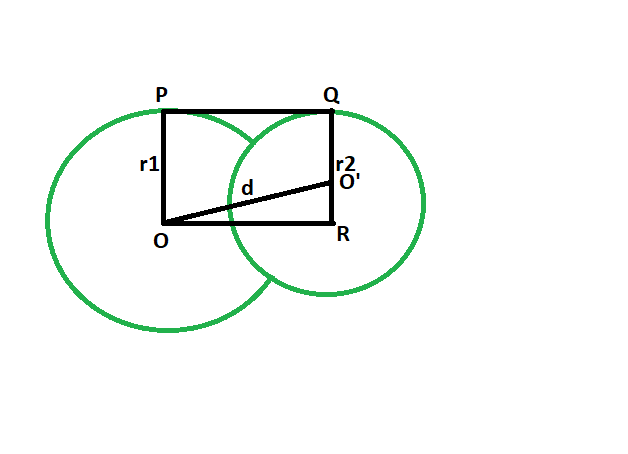# Length of direct common tangent between two intersecting Circles

• Last Updated : 03 Aug, 2022

Given two circles, of given radii, have there centres a given distance apart, such that the circles intersect each other at two points. The task is to find the length of the direct common tangent between the circles.
Examples:

```Input: r1 = 4, r2 = 6, d = 3
Output: 2.23607

Input: r1 = 14, r2 = 43, d = 35
Output: 19.5959```Approach:

• Let the radii of the circles be r1 & r2 respectively.

• Let the distance between the centers be d units.

• Draw a line OR parallel to PQ

• angle OPQ = 90 deg
angle O’QP = 90 deg
{ line joining the centre of the circle to the point of contact makes an angle of 90 degrees with the tangent }

• angle OPQ + angle O’QP = 180 deg
OP || QR

• Since opposite sides are parallel and interior angles are 90, therefore OPQR is a rectangle.

• So OP = QR = r1 and PQ = OR = d

• In triangle OO’R
angle ORO’ = 90
By Pythagoras theorem
OR^2 + O’R^2 = (OO’^2)
OR^2 + (r1-r2)^2 = d^2

• so, OR^2= d^2-(r1-r2)^2
OR = √{d^2-(r1-r2)^2}Below is the implementation of the above approach:

## C++

 `// C++ program to find` `// the length of the direct` `// common tangent between two circles` `// which intersect each other` `#include ` `using` `namespace` `std;`   `// Function to find the length of the direct common tangent` `void` `lengtang(``double` `r1, ``double` `r2, ``double` `d)` `{` `    ``cout << ``"The length of the direct"` `         ``<<``" common tangent is "` `         ``<< ``sqrt``(``pow``(d, 2) - ``pow``((r1 - r2), 2))` `         ``<< endl;` `}`   `// Driver code` `int` `main()` `{` `    ``double` `r1 = 4, r2 = 6, d = 3;` `    ``lengtang(r1, r2, d);` `    ``return` `0;` `}`

## Java

 `// Java program to find` `// the length of the direct` `// common tangent between two circles` `// which intersect each other` `class` `GFG` `{`   `    ``// Function to find the length of ` `    ``// the direct common tangent` `    ``static` `void` `lengtang(``double` `r1, ``double` `r2, ``double` `d) ` `    ``{` `        ``System.out.println(``"The length of the direct"` `                ``+ ``" common tangent is "` `                ``+ (Math.sqrt(Math.pow(d, ``2``) - ` `                    ``Math.pow((r1 - r2), ``2``))));` `    ``}`   `    ``// Driver code` `    ``public` `static` `void` `main(String[] args) ` `    ``{` `        ``double` `r1 = ``4``, r2 = ``6``, d = ``3``;` `        ``lengtang(r1, r2, d);` `    ``}` `}`   `/* This code contributed by PrinciRaj1992 */`

## Python3

 `# Python program to find ` `# the length of the direct ` `# common tangent between two circles ` `# which intersect each other ` ` `  `# Function to find the length of ` `# the direct common tangent ` `def` `lengtang(r1, r2, d): ` `    ``print``(``"The length of the direct common tangent is "` `        ``,((d``*``*` `2``) ``-` `((r1 ``-` `r2)``*``*` `2``))``*``*``(``1``/``2``));`   ` `  `# Driver code` `r1 ``=` `4``; r2 ``=` `6``; d ``=` `3``; ` `lengtang(r1, r2, d); `   `# This code has been contributed by 29AjayKumar`

## C#

 `// C# program to find` `// the length of the direct` `// common tangent between two circles` `// which intersect each other` `using` `System;`   `class` `GFG` `{`   `    ``// Function to find the length of ` `    ``// the direct common tangent` `    ``static` `void` `lengtang(``double` `r1, ``double` `r2, ``double` `d) ` `    ``{` `        ``Console.WriteLine(``"The length of the direct"` `                ``+ ``" common tangent is "` `                ``+ (Math.Sqrt(Math.Pow(d, 2) - ` `                    ``Math.Pow((r1 - r2), 2))));` `    ``}`   `    ``// Driver code` `    ``public` `static` `void` `Main(String[] args) ` `    ``{` `        ``double` `r1 = 4, r2 = 6, d = 3;` `        ``lengtang(r1, r2, d);` `    ``}` `}`   `/* This code contributed by PrinciRaj1992 */`

## PHP

 ``

## Javascript

 ``

Output:

`The length of the direct common tangent is 2.23607`

Time Complexity: O(logn) because suing inbuilt sqrt and pow function

Auxiliary Space: O(1)

My Personal Notes arrow_drop_up
Recommended Articles
Page :